Reach Us+44-1522-440391
Model Tumor Pattern and Compare Treatment Effects Using Semiparametric Linear Mixed-Effects Models | OMICS International
Journal of Biometrics & Biostatistics

Like us on:

All submissions of the EM system will be redirected to Online Manuscript Submission System. Authors are requested to submit articles directly to Online Manuscript Submission System of respective journal.

# Model Tumor Pattern and Compare Treatment Effects Using Semiparametric Linear Mixed-Effects Models

Changming Xia1, Jianrong Wu2 and Hua Liang1*

1Department of Biostatistics and Computational Biology, University of Rochester Medical Center, Rochester, NY 14642, USA

2Department of Biostatistics, St Jude Children’s Research Hospital, Memphis, TN 38105, USA

*Corresponding Author:
Hua Liang
Department of Biostatistics and Computational Biology
University of Rochester Medical Center
Rochester, New York 14642, USA
E-mail: [email protected]

Received Date: May 15, 2013; Accepted Date: June 17, 2013; Published Date: June 21, 2013

Citation: Xia C, Wu J, Liang H (2013) Model Tumor Pattern and Compare Treatment Effects Using Semiparametric Linear Mixed-Effects Models. J Biomet Biostat 4:168. doi:10.4172/2155-6180.1000168

Copyright: © 2013 Xia C, et al. This is an open-access article distributed under the terms of the Creative Commons Attribution License, which permits unrestricted use, distribution, and reproduction in any medium, provided the original author and source are credited.

Visit for more related articles at Journal of Biometrics & Biostatistics

#### Abstract

To analyze responses of solid tumor to treatments and to compare treatment effects with antitumor therapies, we applied semiparametric mixed-effects models to fit tumor volumes measured over a period. The population and individual nonparametric functions were approximated by smoothing spline. We also proposed an intuitive method for a comparison of the antitumor effects of two different treatments. Biological interpretation was also discussed.

#### Keywords

Antitumor xenograft model; Cubic splines; Longitudinal analysis; Semiparametric models; Smoothing spline ANOVA

#### Introduction

In anticancer drug development, demonstrating the antitumor activity of anticancer agents in preclinical animal model is important. Tumor volume is a commonly used endpoint of treatment efficacy in the evaluation of antitumor agents in such a preclinical animal tumor model. Intuitively, tumor volumes of animals treated on different antitumor agents may be used to compare the antitumor activity of the treatments. Appropriate analysis of tumor volume is therefore important in anticancer drug development. Survival analysis based on the tumor growth delay [1-3] is often conducted but it sometimes provides insufficient information or even invalid comparison of two treatments when both survival times are the same but tumor volumes are different. Another endpoint is tumor growth inhibition [2-5] that is generally assessed at a pre-specified time point. These approaches give valid results but can be inefficient because the information at other time points is discarded. An alternative approach is to fit tumor growth curves, such as multivariate analysis, regression modeling [6-8]. More recently, Liang and Sha  applied a parametric nonlinear mixedeffects model [10,11] to analyze changes in tumor volume. To reduce the model’s assumptions and make the methods more general and robust, Liang  proposed a nonparametric method to model tumor volume. Although these approaches use the entire dataset, each has their own limitations. For example, parametric mixed-effects models [13-15] impose strong assumptions on underlying biology mechanisms and might produce coefficients with limited biological relevance , whereas nonparametric mixed-effects models impose no assumptions and may lose useful information when some information is available. In this paper, we suggest a compromised strategy and propose a semiparametric linear mixed-effects (SLM) model to fit tumor volumes.

This research is motivated by the data from a drug combination tumor xenograft study generated in the Pediatric Preclinical Testing Program (PPTP) . In this study, the human rhabdomyosarcoma cell line Rh30 was used to evaluate the therapeutic enhancement for the combination of rapamycin with cytotoxic agents. A total of 140 SCID female mice were used to propagate subcutaneously implanted Rh30 tumors. After tumors grew to a certain size, tumor-bearing mice were randomized into 14different treatment groups with 10 mice per group. Cytotoxic agents were administered at their maximum tolerated dose (MTD), 0.5MTD or 0.63MTD or without concomitant rapamycin treatment. All mice were treated for 6 weeks and followed for another 6 weeks without any treatment. The volume of each tumor is measured at the initiation of the study and weekly up to 12 weeks. Mice were euthanized usually when the tumor volume reaches four times its initial volume, thus resulting in incomplete longitudinal tumor volume data, as shown in Figure 1.

Figure 1: Time has been normalized to [0,1] for computation purposes. A:Control B:Rapamycin C:Vincristine(VCR) MTD D:VCR MTD+Rapamycin E:VCR 0.5MTD F:VCR 0.5MTD+Rapamycin G:Cyclophosphamide (CTX) MTD H:CTX MTD+Rapamycin I:CTX 0.5MTD J:CTX 0.5MTD+Rapamycin K:Cisplatin (CDDP) MTD L:CDDP MTD+Rapamycin M:CDDP 0.63MTD N:CDDP 0.63MTD+Rapamycin.

We would like to establish statistical significance of betweengroup differences in growth profiles and investigate the underlying biology. It is desirable to have interpretable parameters that represent characteristics of the growth curves, such as slope interpreted as the tumor growth rate. We also want the model to be flexible enough to allow different shapes of the curves. As in Figure 1, there is generally an upward/downward trend for a given group, but the growth patterns seem to be non-linear with different patterns among the groups. Straight-line regression models are likely to underfit the data. Polynomial regression or nonlinear models fit the observations better, but coefficient estimates can be sensitive to nonlinearity assumptions that one cannot evaluate robustly from the dataset in hand. In this case, it is reasonable to use a class of semiparametric models that keep the trend modeled parametrically, while letting the rest of the model be driven by data nonparametrically. Thus we may take advantage of both the flexibility of nonparametric models and interpretability and parsimony of parametric models.

We use smoothing spline to fit the nonparametric component, which was initially developed for smooth interpolation . Under statistical context it is more appealing to fit curves that pass near the noisy data, but are not restricted to interpolating exactly. The optimum curve under certain criteria can be found by solving a penalized least squares problem , which is discussed in the later Section. The idea is to find a curve that is a good compromise between fidelity and smoothness. A semiparametric model combining linear predictors and smoothing splines can be written in the form of Linear Mixed Effect models (LME) , which enables utilization of theory and computation power in the field. Subject effects and within-subject variations can also be naturally accounted for under this LME framework.

The paper is organized as follows. In Section 2, the SLM model is reviewed and the detail about constructing corresponding models is given. In Section 3, we apply this method to data from a cancer treatment trial and analyze the results, followed by a conclusion section.

#### Materials and Methods

The general semi-parametric linear mixed-effects model assumes that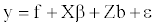(1)

where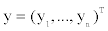are responses;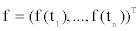is an unknown function of an independent variable t with t∈T and f ∈H, a given Reproducing Kernel Hilbert Space (RKHS), X is the design matrix for some fixed effects with parameters ß , Z is the design matrix for some random effects b,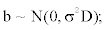and random errors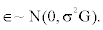H can be decomposed orthogonally as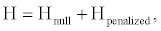(2)

where Hnull is a finite dimensional RKHS spanned by basis functions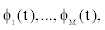and Hpenalized is also a RKHS with reproducing kernel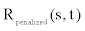Functions in Hnull are not penalized and “preferred” over functions in Hpenalized . For more details on the topic see Aronszajn  and Wahba . The estimate of f can be found by minimizing the following penalized sum of squared errors:(3)

where Ppenalized is the orthogonal projection operator of f from H onto the penalizing space Hpenalized . As a special case, cubic spline penalizes roughness by letting , Ppenalized f = f ′′ so linear functions are not penalized. λ is the smoothing parameter controlling the balance between fidelity to original data and departure from nonpenalizing space . Hnull. λ can be estimated by automatic methods such as Generalized Cross-Validation (GCV), Unbiased Risks (UBR) or Generalized Maximum Likelihood (GML), and it is treated as constant once estimated. The three estimates behave similarly for large sample size . GML is used to estimate the smoothing parameter in this paper.

The function that minimizes (3) has the form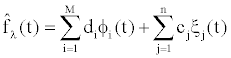(4)

where the coefficients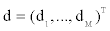and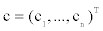are solutions to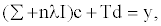(5)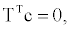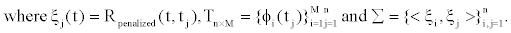A more general form of (3) enables modeling of the covariance matrix using a weight matrix W, and different smoothing components with multiple RKHS decompositions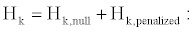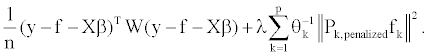(6)

Minimizer to (6) is a simple extension to (4) and (5), and can be directly related [24,25] to the Restricted Maximum Likelihood (REML) solution of the following LME model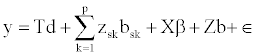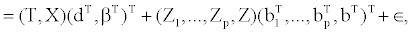(7)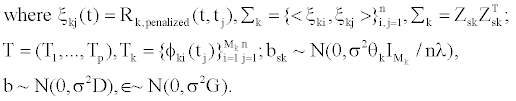Bayesian credible bands are commonly used to evaluate smoothing spline fitted values  by assuming the following prior for f,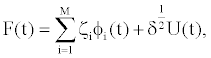(8)

where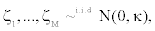U(t) is a zero-mean Gaussian process with covariance function ( , ), penalized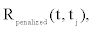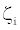and U(t) are independent and κand δ are positive constants. p - values cannot be directly calculated because the distribution (of a function) under the hypothesis is unknown. Such credible bands must be interpreted in an across-the-function fashion, and they cannot be used for describing features of the curve. Since we are estimating a function instead of a real-value parameter, the credible region should represent realizations f (.) of a stochastic process for a fixed time τ. The Bayesian credible bands, however, is based on the average coverage probability (ACP).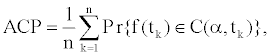(9)

where f (.) is fixed,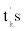are randomly selected and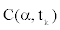t is the corresponding credible band at significance level α . ACP has been shown to be close to nominal coverage rate 1-α in simulations  and theoretical justification . Note that this average coverage is weaker than pointwise coverage.

When comparing two groups with estimated functions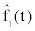and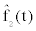, the difference of these two groups can be derived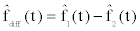with standard error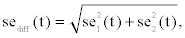where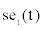and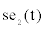are posterior standard errors of the fitted curvesandrespectively. A check for group difference can be performed by examining whether the credible band covers the horizontal zero line. Similar idea was once used in Bowman and Young  and Liang .

As can be seen from (6), the RKHS formulation makes it easy to model parametric and nonparametric components by manipulating the RKHS decomposition, and it combines various spline models under a unified framework. Smoothing spline ANOVA (SS ANOVA) is available with similar interpretation as ordinary ANOVA. Suppose the model space can be decomposed as in (2) and the estimated function is (4) with orthogonal basisand let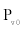be the projection operator onto unpenalized subspace Hnull spanned by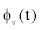for v =1,...,M, and Ppenalized be the projection onto Hpenalized , then the function can be written as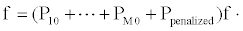(10)

ANOVA and linear regression can be seen as special cases of SS ANOVA by specifying their corresponding decompositions. For an SLM model with linear parametric component and cubic spline nonparametric component, assume the following model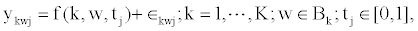where ykwj is the observed tumor volume at time tj of mouse w in group k. For group k, denote Bk as the population from which the mice in group k were drawn and Pk as the sampling distribution. f(k ,w ,tj) is the “true” tumor volume at time tj of mouse w in the population Bk, and k ’s are random errors. f(k ,w ,tj) is a function defined on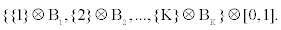Note that f(k ,w ,tj) is a random variable since w is a random sample from Bk. What we observe are realizations of this “true” mean function plus random errors. We use label w to denote mice we actually observe.

We define four averaging operators that project the function f onto modular structures constituting this SLM model: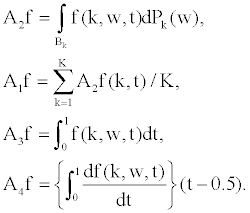Then we have the following SS ANOVA decomposition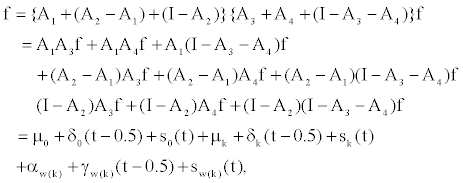(11)

which can be interpreted in parallel with the classical mixed models as follows: μ0 is a constant,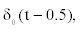is the linear main effect of time,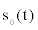is the smooth main effect of time, μk is the main effect of group,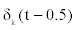is the linear interaction between time and group,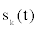is the smooth interaction between time and group,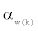is the main effect of mouse,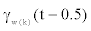is the linear interaction between time and mouse, and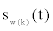is the smooth interaction between time and mouse. We can calculate the main effect of time as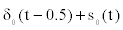, the interaction between time and group as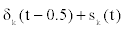and the interaction between time and mouse as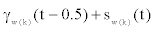. The first six terms are fixed effects. The last three terms are random effects since they depend on the random variable w. Depending on time only, the first three terms represent the mean curve for all mice. The middle three terms measure the departure of a particular group from the population mean curve. The last three terms measure the departure of a particular mouse from the mean curve of a population from which the mouse was chosen.

For categorical variables, we can either estimate each level by shrinkage estimates, which reduce overall means squared error by penalizing departure from overall mean; or we can fit each level separately as fixed categorical covariates. In this study, it is beneficial to not penalize group difference because we are interested in comparing group differences. Both shrinkage and fixed-effects estimates can be fitted under the RKHS framework with different RKHS decompositions. Suppose we want to model time using cubic spline, the categorical variable group as fixed effects and shrink mouse factors toward constants (modeled as random effects), the fixed effects in Equation (11) can be re-written as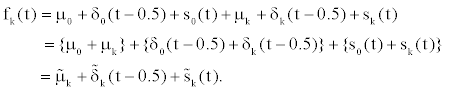(12)

#### Analysis of Xenograft Tumor Data

In this section, we use the proposed method to analyze data from the study described in introduction. Intuitively, the longer mice live, the more favorable the treatment combination is. But the mice with same survival times may have different tumor volumes. The differences in tumor volumes might represent quality of life and reveal potential intervention mechanisms of treatments. For instance, scatterplots from Treatment F and G look quite similar. As we investigate measurements from these two groups, mice with Treatment F tend to die earlier if the tumor volumes are not well controlled at early times, while mice in Group G are more likely to survive after having high initial tumor volumes.

Detail of the data set is given as follows. W=140 mice were assigned to K=14 treatments, and tumor volumes were measured on each mouse weekly for maximum of 12 weeks. Time has been normalized to [0,1]. There are two categorical covariates group and mouse, and a continuous covariate time. We treat group and time as fixed. From the design, the mouse is nested within group and treated as random.

The SLM model discussed in model and methods enables us to (i) estimate the group (treatment) effects; (ii) estimate the population mean volume as functions of time; and (iii) predict response over time for each mouse. For the purpose of this study we are most interested in (i) and (ii).

Based on the SS ANOVA decomposition in (11) and (12), we may fit the following three models. Note that Term 7 in (11), the random effects in intercepts, is not included. From the scatterplots in Figure 1, all mice start out at about the same level (as designed by the study), indicating there is not much of mouse random effects in intercept. Unsurprisingly, the random intercept term causes convergence problems as the nearzero variance is being estimated. Likelihood ratio tests of nested LME objects suggest insignificance of the random intercept beyond the random slope. Therefore, we carry out the analysis without this term.

• Model 1 includes the first six terms and the eighth term in (11). It fits different population mean curves for each group plus a random slope for each mouse. We assume that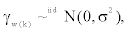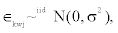and they are mutually independent.

• Model 2 includes all terms except 7th term in (11). It fits different population mean curves for each group plus a random slope and a random smooth effect for each mouse. We assume that’s are stochastic processes which are independent between mice with mean zero and covariance function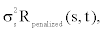withbeing reproducing kernel of the penalized space for cubic splines (specific form can be constructed from scaled recursive Bernoulli polynomials). We further assume within-group errorand independence between the random effects and random errors. These are similar to usual assumptions in LME models.

• Model 3 fits first-order autoregressive correlations structure AR(1) to Model 2, i.e.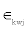’s are no longer assumed to be independent.

• Model 4 uses an extra parameter beyond Model 3 to account for unequal variances of within-group error terms’s by modeling variance as an exponential function of time.

Among all 14 treatments shown in Figure 1, some can be instantly eliminated, such as A and K, because of poor survival times. The analysis is then narrowed down to the 6 groups N, J, F, L, D, G with similar survival times, but may have different tumor volume growth profiles (Figure 2).

Random effects in the smoothing componentscan be fitted manually by constructing a block-diagonal symmetric covariance matrix of dimensions n× n for all observations. Each block corresponds to the reproducing kernelevaluated at observed design points for each subject. Let w =1,...,140, k =1,...,6, observed time points for wth mouse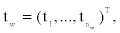random smoothing vector for wth mouse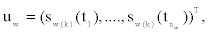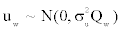where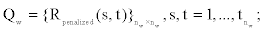total random smoothing vector 1 140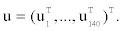.Then random effects in smoothing components can be modeled by specifying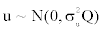, where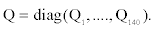Such SLM models are solved by finding solutions of their LME counterparts, as shown in (7). This connection can also be utilized to calculate AIC, BIC and LRT in the sense of conventional parametric models for model selection and comparison, as shown in Table 1. LRTs of these four nested models suggest Model 4 to be most favorable. Estimated serial correlation coefficient for AR(1) in Model 3 is large (0.72), indicating strong within-subject correlation and supporting adequacy of fitting within-subject correlation. Increasing variance over time is quite obvious from scatterplots, which also coincides with the LRT pick of Model 4.

Model AIC BIC logLik Test L.Ratio p-value
1 668.0160 754.6460 -314.0080
2 536.0836 627.0451 -247.0418 1 vs 2 133.9324 <0.0001
3 430.4238 525.7168 -193.2119 2 vs 3 107.6598 <0.0001
4 53.2419 152.8665 -3.62095 3 vs 4 379.1819 <0.0001

Table 1: Model selection criteria for corresponding LME models.

Figure 3 shows predicted curves along with 95% Bayesian credible bands calculated from posterior distributions of fitted values with a diffuse prior by letting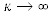in (8). Note that these predicted curves and credible bands are supposed to represent the mean curves for sub-population Bk (Group k), not individuals. This explains why some estimated tumor growth patterns actually start to go down at the end of the study, such as D and F, reflecting the fact that only mice with lower tumor volumes survived among the population of these groups. Figure 4 shows pairwise differences with Bayesian credible bands. If the credible band runs above or below zero, the two treatments are deemed different. Survival times are not under consideration here, so treatment with lower tumor volumes is better (given a mouse survives till that time). For example, treatments N and J are not different from each other since the zero line is fully contained in the credible band. D is better than L because the credible band is mostly above zero. The result is summarized in Table 2.

Figure 4: Pairwise difference estimates with Bayesian credible bands (“-” represents an algebraic minus sign in the top left of each plot).

Pair ANOVA SLM
N-G no no
N-J no no
N-L yes (N) yes (N)
L-J yes (J) yes (J)
N-D yes (D) yes (D)
N-F no yes (F)
L-D yes (D) yes (D)
L-F yes (F) yes (F)
L-G yes (G) yes (G)
J-D yes (D) yes (D)
J-F no yes (F)
J-G no no
F-D yes (D) yes (D)
G-D no yes (D)
G-F yes (G) yes (F)

Table 2: Comparison of the results based on ANOVA and the proposed method-whether difference is detected (the one with lower tumor volumes if detected).

As comparison, result from a simple ANOVA analysis for the logtransformed data is also listed in Table 2. SLM model is not only able to detect more significantly different pairs, but also provides more insight into how the pairs are different from each other. It is worth pointing out that for Treatment G versus Treatment F, although ANOVA and SLM both detect the difference, ANOVA concludes G has lower tumor volumes, while SLM picks F. Simply taking averages in ANOVA ignores information from time and correlation of measurements within each mouse that reveal different behaviors of the two treatment groups towards the end. By looking at the SLM predicted population patterns in Figure 3, while the two tumor growth profiles look similar during early period, tumor volumes in Group F are lower than those in Group G for mice that survived to the final period of the study. For example, if we only look at tumor volumes past normalized time 0.8, the means for Group F and G are 0.4270 and 0.6264 respectively.

#### Remark

The dimension of the random spline covariance matrix Q increases with the number of observations. As a result, direct computation might take a long time. For this specific dataset with all groups, it took about one day to compute the model with random smoothing effects on a personal computer. To speed up the program, a low-rank approximation algorithm was used to reduce the dimension of Q by eliminating eigenvectors corresponding to small eigenvalues . In our example, with a cutoff value 0.001, the approximation reduced the computation time to about an hour and gave almost identical results.

#### Conclusion

SLM model only specifies part of the mixed-effects model parametrically and leaves the rest to be modeled non-parametrically by data itself. It incorporates interpretability and parsimony of a parametric model and flexibility of a nonparametric model, and also avoids estimating parameters with little biological relevance. The close connection with LME models enables us to utilize existing LME fitting procedures for computation and model selection. Even when the final goal is to build a fully parametric model, SLM model can be useful for initial data exploration and shed light on parametric models to follow.

The cost of SLM model is relatively heavy computation (but reasonable with optimization) and difficulty in deriving closed-form inferences in the functional space. More research on model selection methods is needed as well.

Further effort can be invested for some special post-hoc methods such as multiple comparison correction for the SLM model. The major difficulty for such extensions would be establishing an expression of the correction, giving a theoretical justification and calculating p-value.

#### Acknowledgements

The authors gratefully thank two referees for their helpful comments. Liang’s research was partially supported by NSF grants DMS1007167 and DMS1207444 and by Award Number 11228103, given by National Natural Science Foundation of China.

#### References

Select your language of interest to view the total content in your interested language

### Article Usage

• Total views: 12899
• [From(publication date):
October-2013 - Jul 21, 2019]
• Breakdown by view type
• HTML page views : 9072Can't read the image? click here to refresh